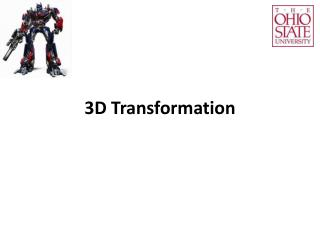DownloadDownload Presentation3D Transformation

# 3D Transformation

Download Presentation## 3D Transformation

- - - - - - - - - - - - - - - - - - - - - - - - - - - E N D - - - - - - - - - - - - - - - - - - - - - - - - - - -
##### Presentation Transcript

1. 3D Transformation

2. 3D Transformation • In 3D, we have x, y, and z. • We will continue use column vectors: . • Homogenous systems: . glVertex3f(x, y ,z);

3. A Right-Handle Coordinate System

4. Transformation: 3D Translation • Given a position (x, y, z) and an • offset vector (tx, ty, tz):

5. Transformation: 3D Scaling • Change the object size:

6. Transformation: 3D Rotation • Rotation needs an angle and an axis. • Rotation is defined according to the right-hand rule (our convention).

7. Transformation: 3D Rotation

8. How to quickly remember them… • The axis coordinate is unchanged. • For the other two coordinates: • Diagonals are filled with cos. • Off-diagonals are filled with sin. • There is a sign…

9. How to quickly remember them… • Here is an easier way to think about it: The vector after rotation The vector before rotation

10. How to quickly remember them… What X becomes After rotation X axis What Y becomes After rotation Y axis What Z becomes After rotation Z axis

11. For example X Y Z Y 1 θ θ X Z

12. Generic Rotation • Use the rotation function: glRotatef(theta, x, y, z); In degree No need to be normalized (x, y, z) Don’t need to remember it, just for your reference. θ

13. How to reverse a transformation • What if I don’t like a transformation, how do I get back?

14. Inverse Translation glTranslatef(tx, ty, tz); glTranslatef(-tx, -ty, -tz);

15. Inverse Scaling glScalef(sx, sy, sz); glScalef(1/sx, 1/sy, 1/sz);

16. Inverse Rotation Rotate_X by θ glRotatef(theta, 1, 0, 0); Rotate_X by -θ glRotatef(-theta, 1, 0, 0);

17. Inverse Transformation • The transformation has multiple matrices: • Its inverse: v v’= Scaling Translation Rotation Translation M1 M2 M3 M4 v v’= Translation Rotation Translation Scaling

18. OpenGL handles multiple matrices glLoadIdentity(); glRotatef(…); glTranslatef(…); glScalef(…); glTranslatef(…); glBegin(GL_POINTS); glVertex3fv(v); glEnd();

19. OpenGL handles multiple matrices Reverse Order • Define v • Translation • Scaling • Translation • Rotation glLoadIdentity(); glRotatef(…); glTranslatef(…); glScalef(…); glTranslatef(…); glBegin(GL_POINTS); glVertex3fv(v); glEnd();

20. OpenGL has a reason. glRotatef(…); //A glTranslatef(…);//B glScalef(…); //C glVertex3fv(v); • Given a world • local1=A(world) • local2=B(local1) • local3=C(local2) • Define v in local3 • Define v • v=Cv • v=Bv • v=Av OpenGL think: world is moved into local. Each transformation is defined respect to the local. We think: v is transformed in a world coordinate system.

21. For example glTranslatef(3,2,0); glVertex3f(2,2,0); OpenGL thinks the coordinate System moves by an offset (3, 2). The vertex defines at (2, 2) locally. We think (2, 2) moves by an offset (3, 2).

22. For example glRotatef(45,0,0,1); glVertex3f(4,0,0); OpenGL thinks the coordinate System rotates 45 degree. The vertex defines at (4, 0) locally. We think (4, 0) rotates 45 degree.

23. For example glRotatef(45,0,0,1); glTranslatef(4,0,0); glVertex3f(0,0,0); We think (0, 0) first Translates, then rotates. OpenGL thinks the coordinate System first rotates, then translates.

24. For example glRotatef(45,0,0,1); glTranslatef(4,0,0); glBegin(…); glVertex3f(0,0,0); glEnd(); L2 Important Note: The second translation is defined respect to L1, not W! L1 W OpenGL thinks the coordinate System first rotates, then translates.

25. L2 A quiz glRotatef(45,0,0,1); glTranslatef(4,0,0); glVertex3f(0,0,0); L1 W L2 glTranslatef(2.8,2.8,0); glRotatef(45,0,0,1); glVertex3f(0,0,0); L1 W (2.8, 2.8)

26. What if we change the order? L2 glRotatef(45,0,0,1); glTranslatef(2.8,2.8,0); glVertex3f(0,0,0); L1 W Important Note: The second transformation is defined respect to L1, not W!

27. Order is important. L2 L2 L1 L1 W W (2.8, 2.8) glTranslatef(2.8,2.8,0); glRotatef(45,0,0,1); glVertex3f(0,0,0); glRotatef(45,0,0,1); glTranslatef(2.8,2.8,0); glVertex3f(0,0,0);

28. A quiz glTranslatef(3,4,0); L1 W (3.0, 4.0)

29. A quiz L2 glTranslatef(3,4,0); L1 glRotatef(45,0,0,1); W (3.0, 4.0)

30. A quiz L3 glTranslatef(3,4,0); L1 glRotatef(45,0,0,1); glScalef(1,2,1); W (3.0, 4.0)

31. L4 A quiz L3 glTranslatef(3,4,0); L1 glRotatef(45,0,0,1); glScalef(1,2,1); W glTranslatef(3,1,0); (3.0, 4.0)

32. L4 A quiz L3 glTranslatef(3,4,0); L1 glRotatef(45,0,0,1); glScalef(1,2,1); W glTranslatef(3,1,0); glVertex3f(3,0,0); (3.0, 4.0)

33. How do I know the coordinate system? L3 OpenGL only use a ModelView matrix M. M is transformed in various ways. M’s effect is to update each vertex as: W

34. How do I know the coordinate system? L3 Case 1: W If we draw a dot at (0, 0, 0) in L3, we actually get (d, h, l) in W.

35. How do I know the coordinate system? Case 2: L3 If we draw a dot at (1, 0, 0) in L3, we actually get (a+d, e+h, i+l) in W. W

36. How do I know the coordinate system? Case 2: L3 W In other words, a unit x vector in L3 is (a, e, i) in W.

37. How do I know the coordinate system? Case 2: L3 W In other words, a unit y vector in L3 is (b, f, j) in W.

38. How do I know the coordinate system? ModelView matrix is the coordinate system L3: L3 L3’s X in W L3’s Z in W W L3’s Origin in W L3’s Y in W

39. Summary • If we think: • Each transformation updates the vertex in an absolute world. • Then, the code is arranged in a reverse order. • Alternatively, OpenGL thinks: • A transformation updates the coordinate system. • For each change, the transformation is defined in the current (local) coordinate system. • Transformation matrix and coordinate system are the same. • The code is in the forward order!